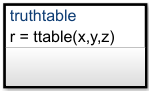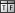## Use Truth Tables to Model Combinatorial Logic

Truth tables implement combinatorial logic design in a tabular format. You can use Stateflow® truth tables to model decision making for fault detection and management and mode switching.

Truth table functions in a Stateflow chart execute only when you call the truth table function. You can define a truth table function in a Stateflow chart, state, or subchart. The location of the function determines the set of states and transitions that can call the function.

• If you want to call the function from within one state or subchart and its substates, put your truth table function in that state or subchart. That function overrides any other functions of the same name in the parents and ancestors of that state or subchart.

• If you want to call the function anywhere in a chart, put your truth table function at the chart level.

• If you want to call the function from any chart in your model, put your truth table at the chart level and enable exporting of chart-level functions. For more information, see Export Stateflow Functions for Reuse.

A truth table function can access chart and state data above it in the Stateflow hierarchy.

Alternatively, for a more direct implementation of decision logic, you can add a Truth Table block directly to your Simulink® model. To implement control logic, Truth Table blocks use MATLAB® as the action language.

### Layout of a Truth Table

This truth table function has the name `ttable`. It takes three arguments (`x`, `y`, and `z`) and returns one output value (`r`).The function consists of this arrangement of conditions, decisions, and actions.

Condition

Decision 1

Decision 2

Decision 3

Decision 4

`x == 1`

T

F

F

-

`y == 1`

F

T

F

-

`z == 1`

F

F

T

-

Action

`r = 1``r = 2``r = 3``r = 4`

Each of the conditions entered in the Condition column must evaluate to true (nonzero value) or false (zero value). Outcomes for each condition are specified as T (true), F (false), or - (true or false). Each of the decision columns combines an outcome for each condition with a logical AND into a compound condition, which is referred to as a decision.

You evaluate a truth table one decision at a time, starting with Decision 1. The Decision 4 covers all possible remaining decisions. If one of the decisions is true, the table perform the associated action, and then the truth table execution is complete.

For example, if conditions `x == 1` and `y == 1` are false and condition `z == 1` is true, then Decision 3 is true and the variable `r` is set equal to 3. The remaining decisions are not tested and evaluation of the truth table is finished. If the first three decisions are false, then the default decision is automatically true and its action (`r=4`) is executed. This table lists pseudocode corresponding to the evaluation of this truth table example.

Pseudocode

Description

```if ((x == 1) & !(y == 1) & !(z == 1)) r = 1; ```

If Decision 1 is true, then set `r=1`.

```elseif (!(x == 1) & (y == 1) & !(z == 1)) r = 2; ```

If Decision 2 is true, then set `r=2`.

```elseif (!(x == 1) & !(y == 1) & (z == 1)) r = 3; ```

If Decision 3 is true, then set `r=3`.

```else r = 4; endif ```

If all other decisions are false, then default decision is true. Set `r=4`.

### Define a Truth Table Function

To define a truth table function:

1. In the object palette, click the truth table function icon.

2. On the chart canvas, click the location for the new truth table function.

3. Enter the signature label for the function.

The signature label of the function specifies a name for your function and the formal names for its arguments and return values. A signature label has this syntax:

``[return_val1,return_val2,...] = function_name(arg1,arg2,...)``
You can specify multiple return values and multiple input arguments. Each return value and input argument can be a scalar, vector, or matrix of values. For functions with only one return value, omit the brackets in the signature label.

You can use the same variable name for both arguments and return values. When you use the same variable for an input and output, you create in-place data. For example, a function with this signature label uses the variables `y1` and `y2` as both inputs and outputs:

`[y1,y2,y3] = f(y1,u,y2)`
If you export this function to C code, the generated code treats `y1` and `y2` as in-place arguments passed by reference. Using in-place data reduces the number of times that the generated code copies intermediate data, which results in more efficient code.

In the Symbols pane and the Model Explorer, the arguments and return values of the function signature appear as data items that belong to your function. Arguments have the scope `Input`. Return values have the scope `Output`.

4. Specify the data properties for each argument and return value, as described in Set Data Properties. When an argument and a return value have the same name, you can edit properties only for the argument. The properties for the return value are read-only.

5. To program the function, open the truth table editor by double-clicking the function box.

6. In the truth table editor, add conditions, decisions, and actions. For more information, see Program a Truth Table.

Your function can access its own data or data belonging to parent states or the chart. The data items in the function can have one of these scopes:

• `Constant` — Constant data retains its initial value through all function calls.

• `Parameter` — Parameter data retains its initial value through all function calls.

• `Local` — Local data persists from one function call to the next function call. Valid only for truth tables that use C as the action language.

• `Temporary` — Temporary data initializes at the start of every function call. Valid only for truth tables that use C as the action language.

In truth table functions that use C as the action language, define local data when you want your data values to persist across function calls throughout the simulation. Define temporary data when you want to initialize data values at the start of every function call. For example, you can define a counter with `Local` scope if you want to track the number of times that you call the function. In contrast, you can designate a loop counter to have `Temporary` scope if you do not need the counter value to persist after the function completes.

In truth table functions that use MATLAB as the action language, you do not need to define local or temporary function data. Instead, in these functions, you can use undefined variables to store values that are accessible only during the rest of the function call. To store values that persist across function calls, use local data at the chart level.

Tip

You can initialize local and temporary data in your function from the MATLAB workspace. For more information, see Initialize Data from the MATLAB Base Workspace.

### Call Truth Table Functions in States and Transitions

You can call truth table functions from the actions of any state or transition or from other functions. If you export a truth table function, you can call it from any chart in the model. For more information about exporting functions, see Export Stateflow Functions for Reuse.

To call a truth table function, use the function signature and include an actual argument value for each formal argument in the function signature.

``[return_val1,return_val2,...] = function_name(arg1,arg2,...)``

If the data types of the actual and formal arguments differ, the function casts the actual argument to the type of the formal argument.

### Specify Properties of Truth Table Functions

The properties listed below specify how a truth table function interacts with the other components in your Stateflow chart. You can modify these properties in the Property Inspector, the Model Explorer, or the Truth Table properties dialog box.

To use the Property Inspector:

1. In the Modeling tab, under Design Data, select .

2. In the Stateflow Editor, select the truth table function.

3. In the Property Inspector, edit the truth table function properties.

To use the Model Explorer:

1. In the Modeling tab, under Design Data, select .

2. In the Model Hierarchy pane, select the truth table function.

3. In the Dialog pane, edit the truth table function properties.

To use the Truth Table properties dialog box:

1. In the Stateflow Editor, right-click the truth table function.

2. Select Properties.

3. In the properties dialog box, edit the truth table function properties.

You can also modify these properties programmatically by using `Stateflow.TruthTable` objects. For more information about the Stateflow programmatic interface, see Overview of the Stateflow API.

#### Name

Function name. Click the function name link to bring your function to the foreground in its native chart.

#### Function Inline Option

Controls the inlining of your function in generated code:

• `Auto` — Determines whether to inline your function based on an internal calculation.

• `Inline` — Inlines your function if you do not export it to other charts and it is not part of a recursion. (A recursion exists if your function calls itself directly or indirectly through another function call.)

• `Function` — Does not inline your function.

This property is not available in the Property Inspector.

#### Label

Signature label for your function. The function signature label specifies a name for your function and the formal names for its arguments and return values. This property is not available in the Property Inspector.

#### Underspecification

Controls the level of diagnostics for underspecification in your truth table function. For more information, see Correct Overspecified and Underspecified Truth Tables.

#### Overspecification

Controls the level of diagnostics for overspecification in your truth table function. For more information, see Correct Overspecified and Underspecified Truth Tables.

#### Action Language

Controls the action language for your Stateflow truth table function. Choose between MATLAB or C. This property is available only In charts that use C as the action language. For more information, see Differences Between MATLAB and C as Action Language Syntax.

#### Description

Description of the truth table function.

Link to online documentation for the truth table function. You can enter a web URL address or a MATLAB command that displays documentation as an HTML file or as text in the MATLAB Command Window. When you click the Document link hyperlink, Stateflow evaluates the link and displays the documentation.

### Specify Properties for Truth Table Blocks

Truth Table block properties specify how your truth table interfaces with the Simulink model. You can modify these properties in the Property Inspector, the Model Explorer, or the Truth Table properties dialog box.

To use the Property Inspector:

1. In the Modeling tab, under Design Data, select .

2. In the Stateflow Editor, click the truth table.

3. In the Property Inspector, edit the truth table properties.

To use the Model Explorer:

1. In the Modeling tab, under Design Data, select .

2. In the Model Hierarchy pane, select the truth table.

3. In the Dialog pane, edit the truth table properties.

To use the Truth Table properties dialog box:

1. Open the Stateflow Editor.

2. In the Modeling tab, click .

3. In the properties dialog box, edit the truth table properties.

You can also modify these properties programmatically by using `Stateflow.TruthTableChart` objects. For more information about the Stateflow programmatic interface, see Overview of the Stateflow API.

Tip

Truth Table block properties are a combination of the properties of truth table functions and charts that use MATLAB as the action language. For a description of each property, see Specify Properties of Truth Table Functions and Specify Properties for Stateflow Charts.